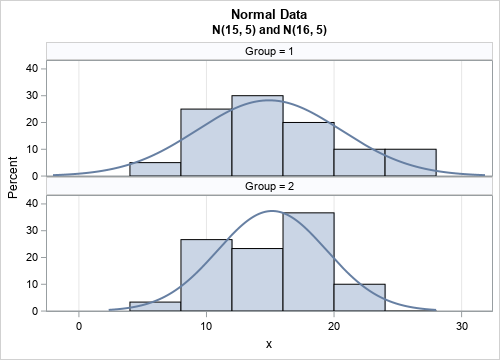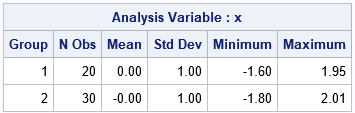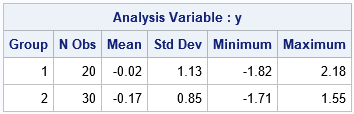A common operation in statistical data analysis is to center and scale a numerical variable. This operation is conceptually easy: you subtract the mean of the variable and divide by the variable's standard deviation. Recently, I wanted to perform a slight variation of the usual standardization:

• Perform a different standardization for each level of a grouping variable.
• Instead of using the sample mean and sample standard deviation, I wanted to center and scale the data by using values that I specify.

Although you can write SAS DATA step code to center and scale the data, PROC STDIZE in SAS contains options that handle both these cases.

### Simulate data from two groups

Let's start by creating some example data. The following DATA step simulates samples drawn from two groups. The distribution of the first group is N(mu1, sigma) and the distribution of the second group is N(mu2, sigma). The first sample size is n1=0; the second size is n2=30. Notice that the data are ordered by the Group variable.

```%let mu1 = 15; %let mu2 = 16; %let sigma = 5; /* Create two samples N(mu1, sigma) and N(mu2, sigma), with samples sizes n1 and n2, respectively */ data TwoSample; call streaminit(54321); n1 = 20; n2 = 30; Group = 1; do i = 1 to n1; x = round(rand('Normal', &mu1, &sigma), 0.01); output; end; Group = 2; do i = 1 to n2; x = round(rand('Normal', &mu2, &sigma), 0.01); output; end; keep Group x; run;   ods graphics / width=500px height=360px; title "Normal Data"; title2 "N(&mu1, &sigma) and N(&mu2, &sigma)"; proc sgpanel data=TwoSample noautolegend; panelby Group / columns=1; histogram x; density x / type=normal; colaxis grid; run;```The graph shows a comparative histogram of the distribution of each group and overlays the density of the normal curve that best fits the data. You can run PROC MEANS to calculate that the estimates for the first group are mean=14.9 and StdDev=5.64. For the second group, the estimates are mean=15.15 and StdDev=4.27.

### The "standard" standardization

Typically, you standardize data by using the sample mean and the sample standard deviation. You can do this by using PROC STDIZE and specify the METHOD=STD method (which is the default method). You can use the BY statement to apply the standardization separately to each group. The following statements standardize the data in each group by using the sample statistics:

```/* use PROC STDIZE to standardize by the sample means and sample std dev */ proc stdize data=TwoSample out=ScaleSample method=std; by Group; var x; run;   proc means data=ScaleSample Mean StdDev Min Max ndec=2; class Group; var x; run;```As expected, each sample now has mean=0 and unit standard deviation. Many multivariate analyses center and scale data in order to adjust for the fact that different variables are measured on different scales. PROC STDIZE has many other options for standardizing data.

### Center and scale by using the DATA step

A second way to standardize the data is to use the DATA step to center and scale each variable and each group. You can overwrite the contents of each column, or (as I've done below), you can create a new variable that contains the standardized values. You need to specify values for the location and scale parameters. For these simulated data, I know the population values of the location and scale parameters. The following DATA step uses the parameters to center and scale the data:

```/* because we know the population parameters, we can use them to center and scale the data */ /* DATA step method */ data ScaleData; array mu (&mu1 &mu2); /* put parameter values into an array */ set TwoSample; y = (x-mu[Group]) / &sigma; run;   proc means data=ScaleData Mean StdDev Min Max ndec=2; class Group; var y; run;```Because I use the population parameters instead of the sample estimates, the transformed variable does not have zero mean nor unit variance.

### Wide form: Use the LOCATION and SCALE statements in PROC STDIZE

You can perform the same calculations by using PROC STDIZE. If you look up the documentation for the METHOD= option on the PROC STDIZE statement, you will see that the procedure supports the METHOD=IN(ds) method, which enables you to read parameters from a data set. I will focus on the LOCATION and SCALE parameters; see the documentation for other options.

You can specify the parameters in "wide form" or in "long form". In wide form, each column specifies a parameter and you can include multiple rows if you want to perform a BY-group analysis. You can use the LOCATION and SCALE statements to specify the name of the variables that contain the parameters. The following DATA step creates a data set that has three variables: Group, Mu, and Sigma. Each row specifies the location and scale parameter for centering and scaling data in the levels of the Group variable.

```data ScaleWide; Group=1; Mu=&mu1; Sigma=&sigma; output; Group=2; Mu=&mu2; Sigma=&sigma; output; run;   proc stdize data=TwoSample out=StdWide method=in(ScaleWide); by Group; var x; location mu; /* column name METHOD=IN data set */ scale sigma; /* column name METHOD=IN data set */ run;   proc means data=StdWide Mean StdDev Min Max ndec=2; class Group; var x; run;```

The output is identical to the output from the previous section and is not shown.

### Long form: Use the _TYPE_ variable

You can also specify the location and scale parameters in long form. For a long-form specification, you need to create a data set that contains a variable named _TYPE_. The parameters for centering and scaling are specified in rows where _TYPE_="LOCATION" and _TYPE_="SCALE", respectively. You can also include one or more BY-group variables (if you are using the BY statement) and one or more columns whose names are the same as the variables you intend to transform.

For example, the example data in this article has a BY-group variable named Group and an analysis variable named X. The following DATA step specifies the values to use to center (_TYPE_='LOCATION') and scale (_TYPE_='SCALE') the X variable for each group:

```data ScaleParam; length _TYPE_ \$14; input Group _TYPE_ x; datalines; 1 LOCATION 15 1 SCALE 5 2 LOCATION 16 2 SCALE 5 ;   proc stdize data=TwoSample out=Scale2 method=in(ScaleParam); by Group; var x; run;   proc means data=Scale2; class Group; var x; run;```

Again, the result (not shown) is the same as when the DATA step is used to center and scale the data.

### Summary

This article gives examples of using PROC STDIZE in SAS/STAT to center and scale data. Most analysts want to standardize data in groups by using the sample means and sample standard deviations of each group. This is the default behavior of PROC STDIZE. However, you can also center and scale data by using values that are different from the group statistics. For example, you might want to use a grand mean and a pooled standard deviation for the groups. Or, perhaps you are using simulated data and you know the population mean and variance of each group. Regardless, there are three ways to center and scale the data when you know the location and scale parameters:

• Manually: You can use the DATA step or PROC IML or PROC SQL to write a formula that centers and scales the data.
• Wide Form: PROC STDIZE supports the LOCATION and SCALE statements. If you use the METHOD=IN(ds) option on the PROC STDIZE statement, you can read the parameters from a data set.
• Long Form: If the input data set contains a variable named _TYPE_, PROC STDIZE will use the parameter values where _TYPE_='LOCATION' to center the data and where _TYPE_='SCALE' to scale the data.
ShareDistinguished Researcher in Computational Statistics

Rick Wicklin, PhD, is a distinguished researcher in computational statistics at SAS and is a principal developer of SAS/IML software. His areas of expertise include computational statistics, simulation, statistical graphics, and modern methods in statistical data analysis. Rick is author of the books Statistical Programming with SAS/IML Software and Simulating Data with SAS.

1.